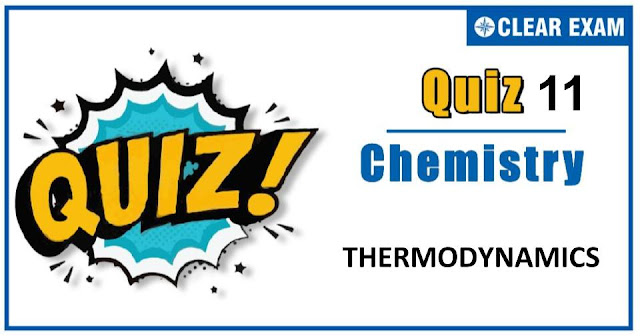## [LATEST]\$type=sticky\$show=home\$rm=0\$va=0\$count=4\$va=0

Thermodynamics is a very important branch of both chemistry and physics. It deals with the study of energy, the conversion of energy between different forms and the ability of energy to do work. Thermodynamics is an important chapter for JEE Mains, JEE Advance NEET and many others exams. As this chapter is present in both chemistry and physics and there is only a minor difference between them hence it becomes more important topic. It is not a tough topic, it only requires practice. This topic has been given good weightage in all engineering exams. So don't skip this and prepare well. All the best !.

Q1. A cylinder of gas is assumed to contain 11.2 kg of butane (C4H10). If a normal family needs 20000 kJ of energy per day, the cylinder will last ( Given that ΔH for combustion of butane is −2658 kJ )
•  20 days
•  25 days
•  26 days
•  24 days
Solution:-
Cylinder contains 11.2kg or 193.10 mole butane. (∵molecular mass of butane = 58)
∵ Energy released by 1 mole of butane = −2658
∵ Energy released by 193.10 mole of butane = −2658 × 193.10
= 5.13 × 105 kJ
∴ 5.13×105 ÷ 20000 = 25.66 or 26 days

Q2. Heat of combustion of CH4, C2H4, C2H6 are -890, -1411 and -1560 kJ/mol respectively. Which has the lowest calorific fuel value in kJ/g ?
•  CH4
•  C2H4
•  C2H6
•  All same
Solution:-
calorific value = Heat of combustion per g of fuel,
i.e. for C2H4, it is −1411÷28, the lowest value.

Q3. 16 g oxygen gas expands at STP to occupy double of its original volume. The work done during the process is :
•  260 kcal
•  180 kcal
•  130 kcal
•  272.8 kcal
Solution:-
At STP, 16g O2 or 1/2 mole O2will occupy 11.2 litre. Thus if volume is doubled, it means
(V2 − V1) = 22.4−11.2 = 11.2 litre
Now, W = P × (V2 − V1) = 1×11.2 litre atm
= (1×11.2×2)÷0.0821 = 272.84 kcal

Q4. When two moles of hydrogen expands isothermally against a constant pressure of 1 atm, at 25 °C from 15L to 50L, the work done (in litre atm) will be :
•  17.5
•  35
•  51.5
•  70
Solution:-
p = 1 atm
ΔV=(50−15)=35L
∴  W = −p.ΔV = −1×35 = −35 atm L
Hence work done by the system on the surroundings is equal to 35 L-atm

Q5. The enthalpy changes of formation of the gaseous oxides of nitrogen(N2O and NO) are positive because of :
•  The high bond energy of the nitrogen molecule
•  The high electron affinity of the oxygen atoms
•  The high electron affinity of the nitrogen atoms
•  The tendency of oxygen to form O2−
Solution:-
Due to high bond energy of N≡N, more heat is absorbed to breakup N2 molecule.

Q6. The enthalpy change is negative for :
•  Cl(g) + aq → Cl(aq)
•  Cl(g) → Cl+(g)+ e
•  1/2 Cl2(g) → Cl(g)
•  Cl2(l) → Cl2(g)
Solution:-
Rest all are endothrmic process. Hydration is always exothermic.

Q7. A carnot engine operates between temperature T and 400K (T>400K). If efficiency of engine is 25%, the temperature T is :
•  400 K
•  500 K
•  533.3 K
•  600 K
Solution:-
ηefficiency=(T2 − T1)/T2, here T2 = T and T1 = 400K
∴ T=533.3 K

Q8. A reaction occur spontaneously if :
•  TΔS = ΔH and both ΔH and ΔS are positive.
•  TΔS > ΔH and both ΔH and ΔS are positive.
•  TΔS < ΔH and both ΔH and ΔS are positive.
•  TΔS > ΔH and ΔH is positive and ΔS is negative.
Solution:-
For spontaneous reaction ΔG = −ve.
ΔG = ΔH − TΔS
ΔH = +ve, ΔS = +ve and TΔS > ΔH

Q9. The resultant heat change in a reaction is same whether it take place in one or several stages. This statement is called
•  Lavoisier and Laplace law
•  Hess's law
•  Joule's law
•  Le-chatelier's principle
Solution:-
Hess's law

Q10. The heat of combustion of ethanol determined by a bomb calorimeter is −670.48 kcal/mol at 25°C. What is ΔU at 25°C for the following reaction ?
C2H5OH(l) + 7/2 O2(g) → 2CO2(g) + 3H2O(g)
•  −335.24 kcal
•  −669.28 kcal
•  −670.48 kcal
•  +670.48 kcal
Solution:-
Experimental determination of heat of reaction by bomb calorimeter represents its value at constant volume i.e. ΔU.## Want to know more

Please fill in the details below:

## Latest NEET Articles\$type=three\$c=3\$author=hide\$comment=hide\$rm=hide\$date=hide\$snippet=hide

Name

ltr
item
BEST NEET COACHING CENTER | BEST IIT JEE COACHING INSTITUTE | BEST NEET, IIT JEE COACHING INSTITUTE: Thermodynamics Quiz-11
Thermodynamics Quiz-11
https://1.bp.blogspot.com/-VWH2_pukI84/X5lyHNdngXI/AAAAAAAAB34/KaMiLNT_6n0nrzpKU_K7K9pusp_vLlmZQCLcBGAsYHQ/w640-h336/Quiz%2BImage%2B20%2B%25287%2529.jpg
https://1.bp.blogspot.com/-VWH2_pukI84/X5lyHNdngXI/AAAAAAAAB34/KaMiLNT_6n0nrzpKU_K7K9pusp_vLlmZQCLcBGAsYHQ/s72-w640-c-h336/Quiz%2BImage%2B20%2B%25287%2529.jpg
BEST NEET COACHING CENTER | BEST IIT JEE COACHING INSTITUTE | BEST NEET, IIT JEE COACHING INSTITUTE
https://www.cleariitmedical.com/2020/10/thermodynamics-quiz-1.html
https://www.cleariitmedical.com/
https://www.cleariitmedical.com/
https://www.cleariitmedical.com/2020/10/thermodynamics-quiz-1.html
true
7783647550433378923
UTF-8